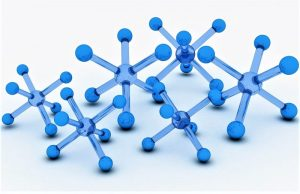Themes

What is the atomic mass?

Atomic mass

The substances that we can observe occupy a space and also have mass . Molecules, which are the constituent parts of substances, often have to be measured through experiments, and it is important that these measurements are accurate. But how can we measure something so small accurately? How do we normally measure molecules?• Symbol:  uo Da
• Unit:   Unit of atomic mass or Dalton

What is the atomic mass?

The atomic mass is the mass that an atom has when it is at rest , it is the one that arises from the total mass of protons and neutrons of a single atom also at rest.

In different laboratories , a tool called an analytical balance is used to measure in grams . Through careful calculation, scientists can determine how many moles a specific reaction will require. A mole is a unit of measurement that helps us make comparisons between particles of any given substance and their mass . If we already know how many moles we need, we can use the concept of molar mass to calculate how many grams of the substance are required. The molar mass, also known as molecular weight, is the sum of the total mass in grams of all the atoms that make up a particular molecule . The unit used to measure is grams per mole.

• History
• What does the atomic mass represent
• How it is represented
• How to measure atomic mass
• How atomic mass is calculated
• Examples

History

The first scientist to study atomic weight and to calculate it was the English chemist John Dalton . He has been known for having developed a theory in this sense that was based on five fundamental points. In addition to John Dalton, Jöns Jakob Berzelius , a Swedish chemist who also performed various calculations and who, along with the former scientist, is considered the father of modern chemistry . Polymer , catalysis or isomer were some of the concepts that he coined and that he introduced in this science.

What does the atomic mass represent

The atomic mass represents the amount of different isotopes that can be found in the same element, but that differ from each other and is at the same time one of the fundamental properties that atoms have, such as number and mass. Sometimes we find that there is an isotope that is more common than others, and the difference between its atomic mass and the relative atomic mass or standard atomic weight is small enough that it does not affect the calculations, but this difference may be important in other cases.

How it is represented

The atomic mass of the elements is represented in the periodic table of the elements by means of the letter A.  It is represented by means of the mass number and this number is the one that indicates the total number of particles that can be found in the nucleus. , in other words the sum and of the neutrons and of the protons . It is responsible for representing the mass of the atom measured in amu.On some occasions it is also known by the name of atomic weight , however, it is important to mention that this name is incorrect since mass is a specific property of the bodyOn the other hand, the weight is a characteristic that will depend entirely on the weight .

How to measure atomic mass

The unit in which the atomic mass is measured is precisely called the atomic mass unit and is represented by the acronym uma . This unit is also called Dalton , and is represented by the word Da, which was given in honor of the chemist John Dalton . The amu corresponds to one twelfth of the mass of the nucleus of carbon-12, which is the most abundant isotope of this element. The value of an amu is also very close to the mass of a proton . Measuring the masses of the elements it can be performed by a spectrometer of mass. The scientific unit for handling atoms in macroscopic quantities is known as the mole .

How atomic mass is calculated

Atomic mass can be calculated by adding the numbers of neutrons and the protons or electrons of a given element. They can also be calculated with the weighted measures of the masses of the different isotopes that make up each element.

Examples

• Atomic mass of oxygen:  In the case of hydrogen, it indicates atomic mass of H = 1.00797, we must read that the mass of an atom of H is 1.00797 times greater than the amu, but not that the mass of an atom of H is 1.00797 g.
• Atomic mass of carbon:  Natural carbon is a mixture of three isotopes, 98.892% of 12 C and 1.108% of 13 C and a minimum amount of 14 C. Therefore, the average atomic mass of carbon will be: (0.98892 ) x (12 amu) + (0.01108) x (13.00335 amu) = 12.011 amu
• Some other atomic masses are:  Calcium 40.087, Cobalt 58.933195, Copper 63.564, Fluorine 18.998403 and Iron 55.845.